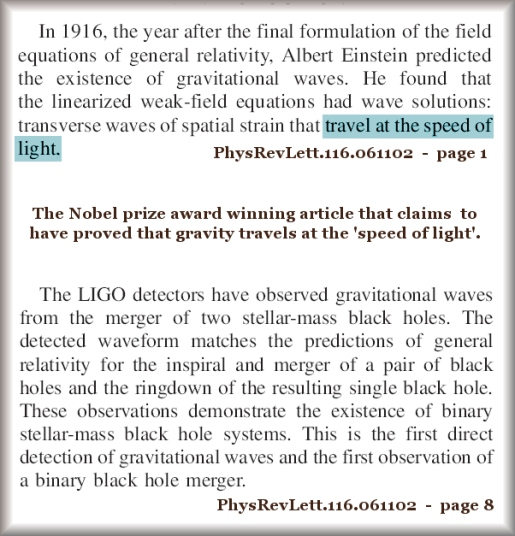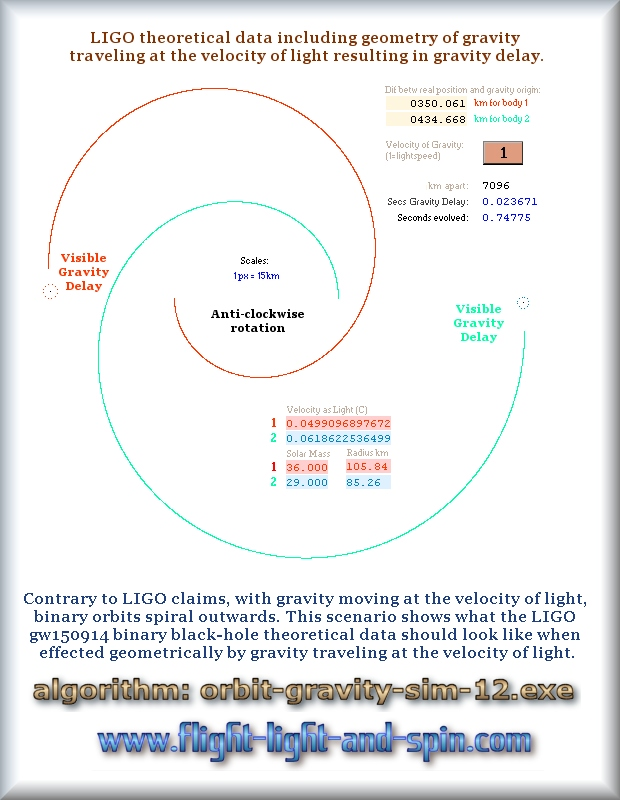What is the Speed of Gravity?

 Since at least the time of Newton, theorists have wondered whether gravity is an instant force, or if gravity travels at the speed of light. Einstein's theory of General Relativity claims to have predicted that 'gravity travels at the speed of light'. The aim of the LIGO experiments was to prove this. Regardless of the validity of any theory, there are many online reports that have failed to realize this basic point in the history of science as to what the claims actually are. Such reports will have it that Relativity does not claim that gravity travels at any speed. But the theory does indeed claim that 'gravity travels at the speed of light'. Quantum Mechanics and Relativity both have in common, the idea that 'gravity travels at the speed of light'. Link to: Video of this articleIt is quite clear that Hawking reports that gravity travels at the speed of light. The Nobel Prize was awarded in 2017 to the LIGO group which claim to have proved that gravity is a 'wave' and that such gravity 'travels at the speed of light.'However, when applying the principle that 'gravity travels at the speed of light' to a computer algorithm depicting the orbits of an ordinary binary star system - specifically the binary pair known as 'Alpha Centauri' - the result is that gravity is delayed by between 1.5 hours and 5 hours in order to travel the varying distance between the binary stars.

 This shows that if gravity 'travels at the speed of light', then the Alpha Centauri binary would separate by more than 1.4 million km per orbit. After 50 orbits, the maximum distance would be about 70 mkm more than the maximum for the first orbit. This is visible on the scale of the computer screen (above image) as the orbit line gets wider by about 5 pixels on that scale. The algorithm that evolved that orbital graphic is freely available at the links at the bottom of the page. The result would be that such an outwards spiral would increase the orbit of the Alpha Centauri binary - from a distance apart the same as from Mercury to the Sun - to a distance apart equal to that from Pluto to the Sun, in one third of a million years. (See reference at the end of this article)*If gravity is instantly propagated then it pulls directly through the midpoint returning the binary pair to their origins after each orbit. But if 'gravity travels at the speed of light' then it pulls to a direction behind the midpoint due to the delay. Here it pulls to where the opposite star was, not where it now is at the time of the gravity arriving at the other star. This changes the direction of the force and thus it must also change the shape of the orbit considerably.The Nobel winners have neglected to notice the geometrical consequence of gravity traveling at the velocity of light - when applied to ordinary star systems, and basic planetary systems. A delay in the effect of gravity would cause the moon to separate from the Earth, at more than 400m per orbit* and most other planets to separate from the Sun - if gravity travels at the velocity of light. This is catastrophic evidence against gravity having any velocity at all, and thus it must be concluded that gravity is an instantaneous field. Moreover, if nothing that travels at the velocity of light can escape a black-hole - and gravity is said to be traveling at the velocity of light - then it would follow that the 'gravitational-wave' would not be able to escape the 'curved space' of the black-hole. If light cannot escape a black-hole, then gravity cannot escape a black-hole either - if they have the same velocity. The black-hole should give off zero gravity as a point of sheer logic. Thus black-holes would not be able to form a binary system which requires gravity to be transmitted between the pair to keep them in orbit around each other. The entire thesis of Relativity, and that of the LIGO experiments is based on numerous points of logical error. However, that does not mean that the LIGO detector is bad or false technology; far from it. But it does mean that their explanation in terms of theoretical physics is just plain wrong. Video of this: https://youtu.be/xNJ7jRIBPjAThis has been a brief summary. A more precise analysis can be found in the more detailed articles to follow. These articles yield several main discoveries that are more than a mere paradigm-shift in Astrophysics. Those results need to be looked at in their complete detail, in order to be fully appreciated. Here are those more expansive articles:
 1} Gravitational Waves and General Relativity A critical analysis in natural philosophy 2} Analysis of GW150914 Includes algorithm orbit-gravity-sim-11.exe *3} Relativity and the Solar System Includes algorithm orbit-gravity-sim-12.exe

Jonathan Ainsley Bain
South Africa, March 30, 2018 A.D.